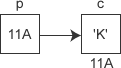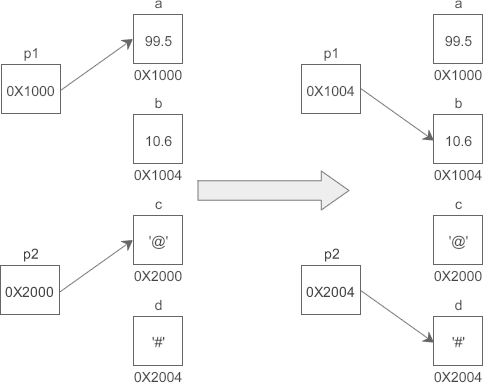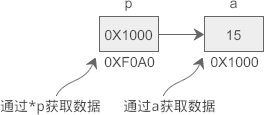# C语言指针变量的定义和使用（精华）## 定义指针变量

datatype *name;

datatype *name = value;

`*`表示这是一个指针变量，`datatype`表示该指针变量所指向的数据的类型 。例如：
`int *p1;`
p1 是一个指向 int 类型数据的指针变量，至于 p1 究竟指向哪一份数据，应该由赋予它的值决定。再如：
```int a = 100;
int *p_a = &a;```

```//定义普通变量
float a = 99.5, b = 10.6;
char c = '@', d = '#';
//定义指针变量
float *p1 = &a;
char *p2 = &c;
//修改指针变量的值
p1 = &b;
p2 = &d;```
`*`是一个特殊符号，表明一个变量是指针变量，定义 p1、p2 时必须带`*`。而给 p1、p2 赋值时，因为已经知道了它是一个指针变量，就没必要多此一举再带上`*`，后边可以像使用普通变量一样来使用指针变量。也就是说，定义指针变量时必须带`*`，给指针变量赋值时不能带`*``int *a, *b, *c;  //a、b、c 的类型都是 int*`

`int *a, b, c;`

## 通过指针变量取得数据

*pointer;

```#include <stdio.h>

int main(){
int a = 15;
int *p = &a;
printf("%d, %d\n", a, *p);  //两种方式都可以输出a的值
return 0;
}```

15, 15```#include <stdio.h>

int main(){
int a = 15, b = 99, c = 222;
int *p = &a;  //定义指针变量
*p = b;  //通过指针变量修改内存上的数据
c = *p;  //通过指针变量获取内存上的数据
printf("%d, %d, %d, %d\n", a, b, c, *p);
return 0;
}```

99, 99, 99, 99

*p 代表的是 a 中的数据，它等价于 a，可以将另外的一份数据赋值给它，也可以将它赋值给另外的一个变量。

`*`在不同的场景下有不同的作用：`*`可以用在指针变量的定义中，表明这是一个指针变量，以和普通变量区分开；使用指针变量时在前面加`*`表示获取指针指向的数据，或者说表示的是指针指向的数据本身。

```int *p = &a;
*p = 100;```

```int *p;
p = &a;
*p = 100;```

```int x, y, *px = &x, *py = &y;
y = *px + 5;  //表示把x的内容加5并赋给y，*px+5相当于(*px)+5
y = ++*px;  //px的内容加上1之后赋给y，++*px相当于++(*px)
y = *px++;  //相当于y=*(px++)
py = px;  //把一个指针的值赋给另一个指针```

【示例】通过指针交换两个变量的值。
```#include <stdio.h>

int main(){
int a = 100, b = 999, temp;
int *pa = &a, *pb = &b;
printf("a=%d, b=%d\n", a, b);
/*****开始交换*****/
temp = *pa;  //将a的值先保存起来
*pa = *pb;  //将b的值交给a
*pb = temp;  //再将保存起来的a的值交给b
/*****结束交换*****/
printf("a=%d, b=%d\n", a, b);
return 0;
}```

a=100, b=999
a=999, b=100

## 关于 * 和 & 的谜题

`*&a`可以理解为`*(&a)``&a`表示取变量 a 的地址（等价于 pa），`*(&a)`表示取这个地址上的数据（等价于 *pa），绕来绕去，又回到了原点，`*&a`仍然等价于 a。

`&*pa`可以理解为`&(*pa)``*pa`表示取得 pa 指向的数据（等价于 a），`&(*pa)`表示数据的地址（等价于 &a），所以`&*pa`等价于 pa。

## 对星号`*`的总结

• 表示乘法，例如`int a = 3, b = 5, c;  c = a * b;`，这是最容易理解的。
• 表示定义一个指针变量，以和普通变量区分开，例如`int a = 100;  int *p = &a;`
• 表示获取指针指向的数据，是一种间接操作，例如`int a, b, *p = &a;  *p = 100;  b = *p;`